##### How many ways we can load the related entities in Entity Framework?

First of all, we need to understand what is related or child entities in the Entity Framework. For a better understanding, please have a look at the following Student Entity. Inside this Student Entity, you can see, we are having three navigation properties i.e. Standard, StudentAddress as Reference Navigation Property, and Courses as Collection Navigation Property. These Navigation properties are nothing but point to some other Entities. So, related entities ae nothing but entities that are created using Foreign Keys. These other entities are nothing the related entities of the Student Entity.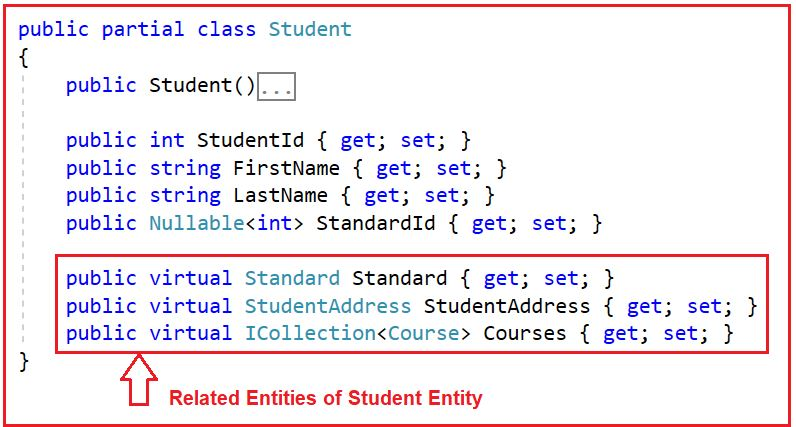Eager loading is a Process where Entity Framework loads the related entities along with the main entity. That means, in this case, Entity Framework will not execute separate SQL queries for loading the related entities. So, all the entities are loaded from the database with a single query and hence saving bandwidth and server CPU time. Entity Framework uses JOINs to load the related entities.

1. Include(string path): This method is used to specify the related objects to include in the query results. Here, the parameter path specifies the dot-separated list of related objects to return in the query results. Here, paths are all-inclusive. For example, if an include call indicates Include(“Standard.Teachers“), not only will Teachers be included, but also Standard.
##### Example to Understand Eager Loading in Entity Framework using LINQ Query Syntax:

What our requirement is, when loading the Students entities, we also need to eager-load the corresponding Standards entities. The students and their Standards will be retrieved in a single query using the SQL join. In the following example, we use LINQ Query Syntax to fetch all the students from the Student database tab;e along with its standards using the DbSet Include() method.

```using System;
using System.Linq;
namespace DBFirstApproach
{
class Program
{
static void Main(string[] args)
{
using (EF_Demo_DBEntities context = new EF_Demo_DBEntities())
{
//To log the generated SQL in the Console
context.Database.Log = Console.Write;

//Include(string path): Specifies the related objects to include in the query results.
//path: The dot-separated list of related objects to return in the query results.

//Method Synatx
var students = context.Students.Include("Standard").ToList();

//Querey Syntax
//var students = (from s in context.Students.Include("Standard")
//                select s).ToList();

//Printing all the Student and Standard details
foreach (var student in students)
{
Console.WriteLine(\$"Firstname: {student.FirstName}, Lastname: {student.LastName}, StandardName: {student.Standard.StandardName}, Description: {student.Standard.Description}");
}

}
}
}
}```

Now, run the above code and you should get the following output. In the output you can see, it is generating a Single SELECT Statement using the SQL JOINs to Fetch the data from both Student and Standard database tables. So, with the Include method or Eager Loading, we can reduce the number of round trips or SQL Statements to be generated and executed on the database server which will improve the overall application performance.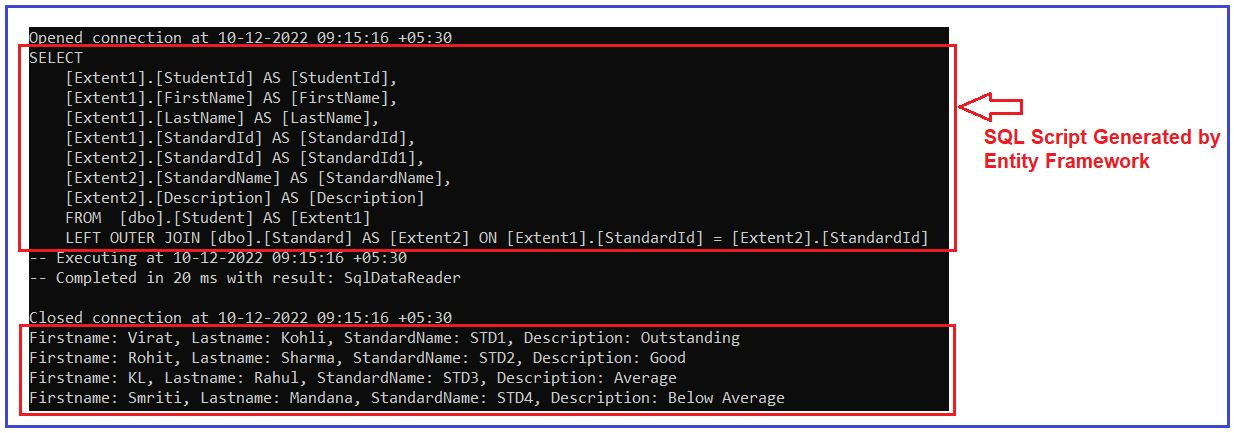We can also use the LINQ Lambda expression as a parameter in the Include method to specify the related entities. For this, first of all, you need to add a reference to System.Data.Entity namespace and then you can use the lambda expression to specify the related properties of an Entity as shown in the below example. This is the same example as the previous one. Here, instead of using a string to specify the related entity, we are using a lambda expression to specify the related entity. You can only specify those entities which are created as Navigation Entities inside the Main entity.

```using System;
using System.Linq;
using System.Data.Entity;
namespace DBFirstApproach
{
class Program
{
static void Main(string[] args)
{
using (EF_Demo_DBEntities context = new EF_Demo_DBEntities())
{
//To log the generated SQL in the Console
context.Database.Log = Console.Write;

//Include(): Specifies the related objects to include in the query results.

//Method Synatx
var students = context.Students.Include(x => x.Standard).ToList();

//Querey Syntax
//var students = (from s in context.Students.Include(x => x.Standard)
//                select s).ToList();

//Printing all the Student and Standard details
foreach (var student in students)
{
Console.WriteLine(\$"Firstname: {student.FirstName}, Lastname: {student.LastName}, StandardName: {student.Standard.StandardName}, Description: {student.Standard.Description}");
}

}
}
}
}```

Now, run the above code and you will get the same output as the previous example. In the output you can also verify, it is generating the same SELECT SQL Statement with JOINs to Fetch the data from both Student and Standard database tables. So, from a performance point of view, there is no difference between both approaches.##### How to Load Multiple Related Entities using Include Method in Entity Framework?

If you look at the following Student Entity, then you will see that this Student Entity Has three related entities as Standard, StudentAddress as Reference Navigational Entities, and Courses as Collection Navigation Entity.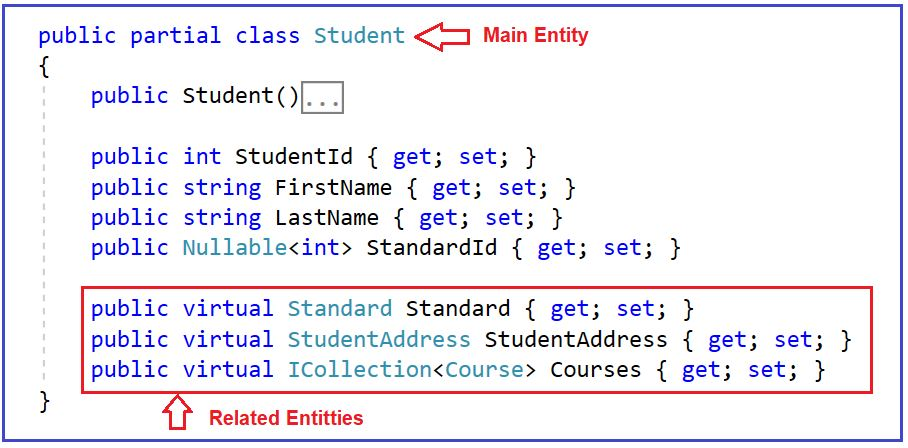Now, our requirement is we want to load the Standard, StudentAddreess, and Courses Entities while loading the Student Entity. Is it possible in Entity Framework? Yes, it is possible. We can eagerly load multiple related entities using the Include method in Entity Framework. In the below example, we eagerly load the Student, Standard, StudentAddress, and Courses entities while loading the Student Entity.

```using System;
using System.Linq;
using System.Data.Entity;
namespace DBFirstApproach
{
class Program
{
static void Main(string[] args)
{
using (EF_Demo_DBEntities context = new EF_Demo_DBEntities())
{
//To log the generated SQL in the Console
context.Database.Log = Console.Write;

//Method Synatx
var students = context.Students
.Include(x => x.Standard)
.Include(x => x.Courses)
.ToList();

//Querey Syntax
//var students = (from s in context.Students
//                 .Include(x => x.Standard)
//                 .Include(x => x.Courses)
//                 select s).ToList();

//Printing all the Student and Standard details
foreach (var student in students)
{
foreach (var course in student.Courses)
{
Console.WriteLine(\$"\tCourse ID: {course.CourseId} Course Name: {course.CourseName}");
}
}

}
}
}
}```

Now, run the application and you should get the Student and all the related entities data as expected as shown in the below image.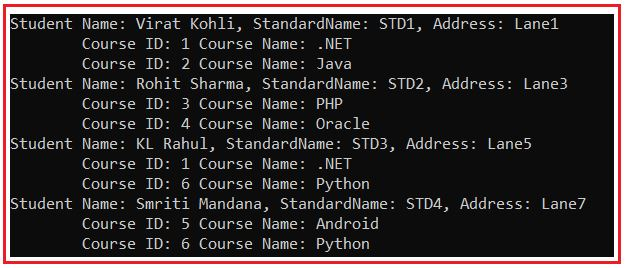Here, you can observe the generated SQL Part and you can see, it is generating a single SELECT SQL Statement by joining with all the related tables as follows:

```SELECT
[Project1].[StudentId1] AS [StudentId],
[Project1].[StudentId] AS [StudentId1],
[Project1].[FirstName] AS [FirstName],
[Project1].[LastName] AS [LastName],
[Project1].[StandardId] AS [StandardId],
[Project1].[StandardId1] AS [StandardId1],
[Project1].[StandardName] AS [StandardName],
[Project1].[Description] AS [Description],
[Project1].[Mobile] AS [Mobile],
[Project1].[Email] AS [Email],
[Project1].[C1] AS [C1],
[Project1].[CourseId] AS [CourseId],
[Project1].[CourseName] AS [CourseName],
[Project1].[TeacherId] AS [TeacherId]
FROM ( SELECT
[Extent1].[StudentId] AS [StudentId],
[Extent1].[FirstName] AS [FirstName],
[Extent1].[LastName] AS [LastName],
[Extent1].[StandardId] AS [StandardId],
[Extent2].[StudentId] AS [StudentId1],
[Extent2].[Mobile] AS [Mobile],
[Extent2].[Email] AS [Email],
[Extent3].[StandardId] AS [StandardId1],
[Extent3].[StandardName] AS [StandardName],
[Extent3].[Description] AS [Description],
[Join3].[CourseId1] AS [CourseId],
[Join3].[CourseName] AS [CourseName],
[Join3].[TeacherId] AS [TeacherId],
CASE WHEN ([Join3].[StudentId] IS NULL) THEN CAST(NULL AS int) ELSE 1 END AS [C1]
FROM    [dbo].[Student] AS [Extent1]
LEFT OUTER JOIN [dbo].[StudentAddress] AS [Extent2] ON [Extent1].[StudentId] = [Extent2].[StudentId]
LEFT OUTER JOIN [dbo].[Standard] AS [Extent3] ON [Extent1].[StandardId] = [Extent3].[StandardId]
LEFT OUTER JOIN  (SELECT [Extent4].[StudentId] AS [StudentId], [Extent5].[CourseId] AS [CourseId1], [Extent5].[CourseName] AS [CourseName], [Extent5].[TeacherId] AS [TeacherId]
FROM  [dbo].[StudentCourse] AS [Extent4]
INNER JOIN [dbo].[Course] AS [Extent5] ON [Extent4].[CourseId] = [Extent5].[CourseId] ) AS [Join3] ON [Extent1].[StudentId] = [Join3].[StudentId]
)  AS [Project1]
ORDER BY [Project1].[StudentId1] ASC, [Project1].[StudentId] ASC, [Project1].[StandardId1] ASC, [Project1].[C1] ASC```
##### How to Load Multiple Levels of Related Entities using the Include Method in Entity Framework?

It is also possible to eagerly load multiple levels of related entities using the Include method in Entity Framework. So, first here, we need to understand what it means by multiple levels of related entities. If you go to the Student entity or Student class, then you will see that it includes one related entity called Standard i.e. it includes it as a Navigation property. Now, if you go to the Standard entity or Standard class, then you will see that it includes one related entity called Teachers i.e. the Standard Entity includes the Teachers entity as a collection navigation entity. Now, what we want is, we want to load the Student, Standard, and Teacher entities. This is nothing but multiple levels of related entities in Entity Framework.

To load multiple levels of related entities, we need to specify the related entities using the dot (.) operation inside the DbSet Include method. In the following example, we are eagerly loading the Student, Standard, and Teacher Entities.

```using System;
using System.Linq;
using System.Data.Entity;
namespace DBFirstApproach
{
class Program
{
static void Main(string[] args)
{
using (EF_Demo_DBEntities context = new EF_Demo_DBEntities())
{
//To log the generated SQL in the Console
context.Database.Log = Console.Write;

//Method Synatx using String Name
var studentsMS = context.Students.Include("Standard.Teachers").ToList();

//Method Synatc Using Lambda Expression
//var studentsMS = context.Students.Include(s => s.Standard.Teachers).ToList();

//Query Syntax using string name
//var studentsQS = (from s in context.Students
//                .Include("Standard.Teachers")
//                select s).ToList();

//Query Syntax using Lambda Expression
//var studentsQS = (from s in context.Students
//                .Include(s => s.Standard.Teachers)
//                  select s).ToList();

//Printing all the Student and Standard details
foreach (var student in studentsMS)
{
Console.WriteLine(\$"Firstname: {student.FirstName}, Lastname: {student.LastName}, StandardName: {student.Standard.StandardName}, Description: {student.Standard.Description}");

//You can also access the Teacher collection here
foreach (var teacher in student.Standard.Teachers)
{
Console.WriteLine(\$"\tTeacher ID: {teacher.TeacherId}, Name: {teacher.FirstName} {teacher.LastName}");
}
}

}
}
}
}```

Now, run the application and you should get the Student and all the multiple levels of related entities data as expected as shown in the below image.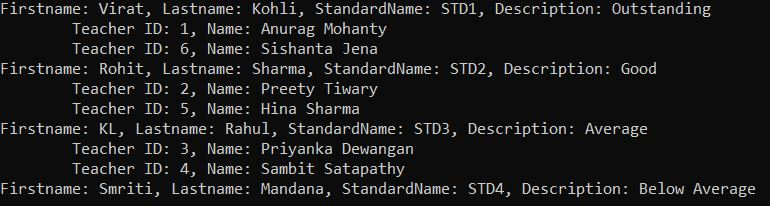Here, you can observe the generated SQL code and you can see, it is generating a single SELECT SQL Statement by joining with all the related tables as follows:

```SELECT
[Project1].[StudentId] AS [StudentId],
[Project1].[FirstName] AS [FirstName],
[Project1].[LastName] AS [LastName],
[Project1].[StandardId] AS [StandardId],
[Project1].[StandardId1] AS [StandardId1],
[Project1].[StandardName] AS [StandardName],
[Project1].[Description] AS [Description],
[Project1].[C1] AS [C1],
[Project1].[TeacherId] AS [TeacherId],
[Project1].[FirstName1] AS [FirstName1],
[Project1].[LastName1] AS [LastName1],
[Project1].[StandardId2] AS [StandardId2]
FROM ( SELECT
[Extent1].[StudentId] AS [StudentId],
[Extent1].[FirstName] AS [FirstName],
[Extent1].[LastName] AS [LastName],
[Extent1].[StandardId] AS [StandardId],
[Extent2].[StandardId] AS [StandardId1],
[Extent2].[StandardName] AS [StandardName],
[Extent2].[Description] AS [Description],
[Extent3].[TeacherId] AS [TeacherId],
[Extent3].[FirstName] AS [FirstName1],
[Extent3].[LastName] AS [LastName1],
[Extent3].[StandardId] AS [StandardId2],
CASE WHEN ([Extent3].[TeacherId] IS NULL) THEN CAST(NULL AS int) ELSE 1 END AS [C1]
FROM   [dbo].[Student] AS [Extent1]
LEFT OUTER JOIN [dbo].[Standard] AS [Extent2] ON [Extent1].[StandardId] = [Extent2].[StandardId]
LEFT OUTER JOIN [dbo].[Teacher] AS [Extent3] ON [Extent3].[StandardId] = [Extent1].[StandardId]
)  AS [Project1]
ORDER BY [Project1].[StudentId] ASC, [Project1].[StandardId1] ASC, [Project1].[C1] ASC```
##### Example to Understand Eagerly Loading one Entity with its Related Data:

In the following example, we Load one Student and its related address and Standard.

```using System;
using System.Linq;
using System.Data.Entity;
namespace DBFirstApproach
{
class Program
{
static void Main(string[] args)
{
using (EF_Demo_DBEntities context = new EF_Demo_DBEntities())
{
//To See what SQL Generated by Entity Framework
context.Database.Log = Console.Write;

var student = context.Students
.Include("Standard")
.FirstOrDefault(s => s.StandardId == 1);

}
}
}
}```
###### Output: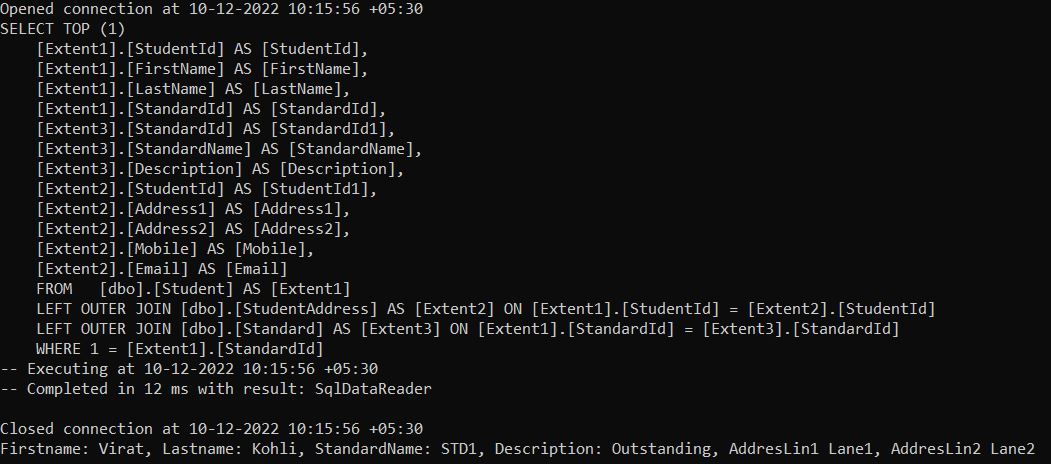1.